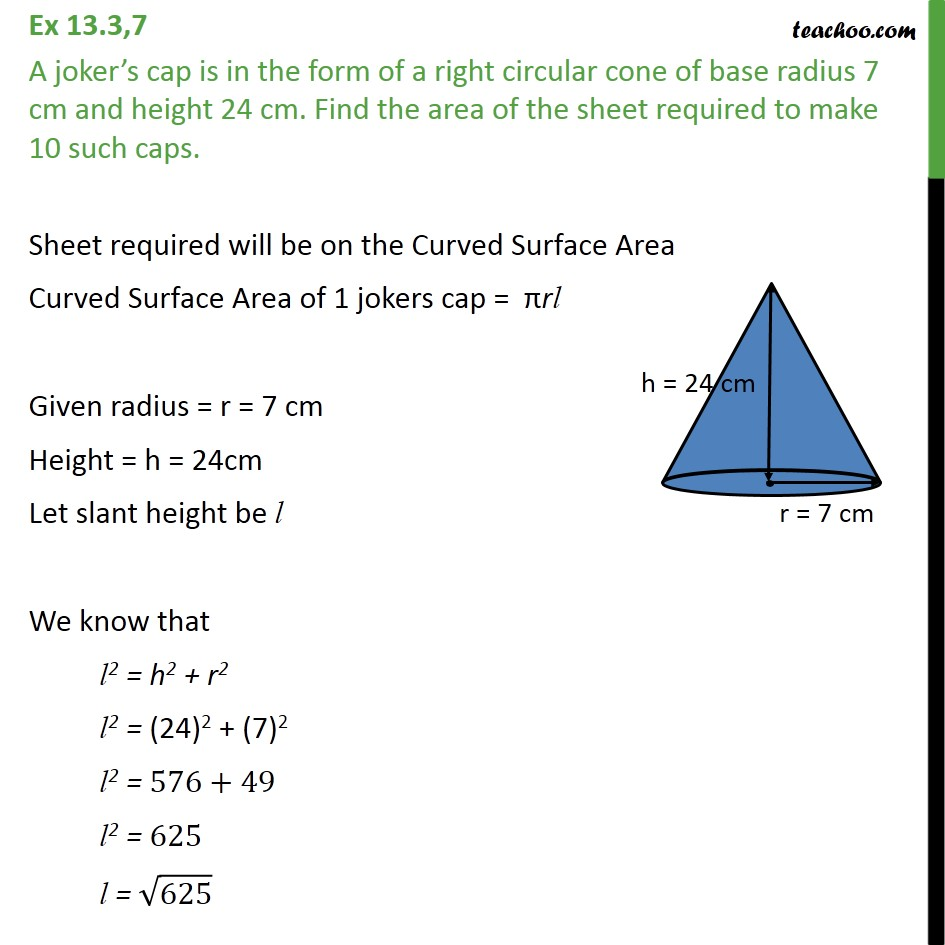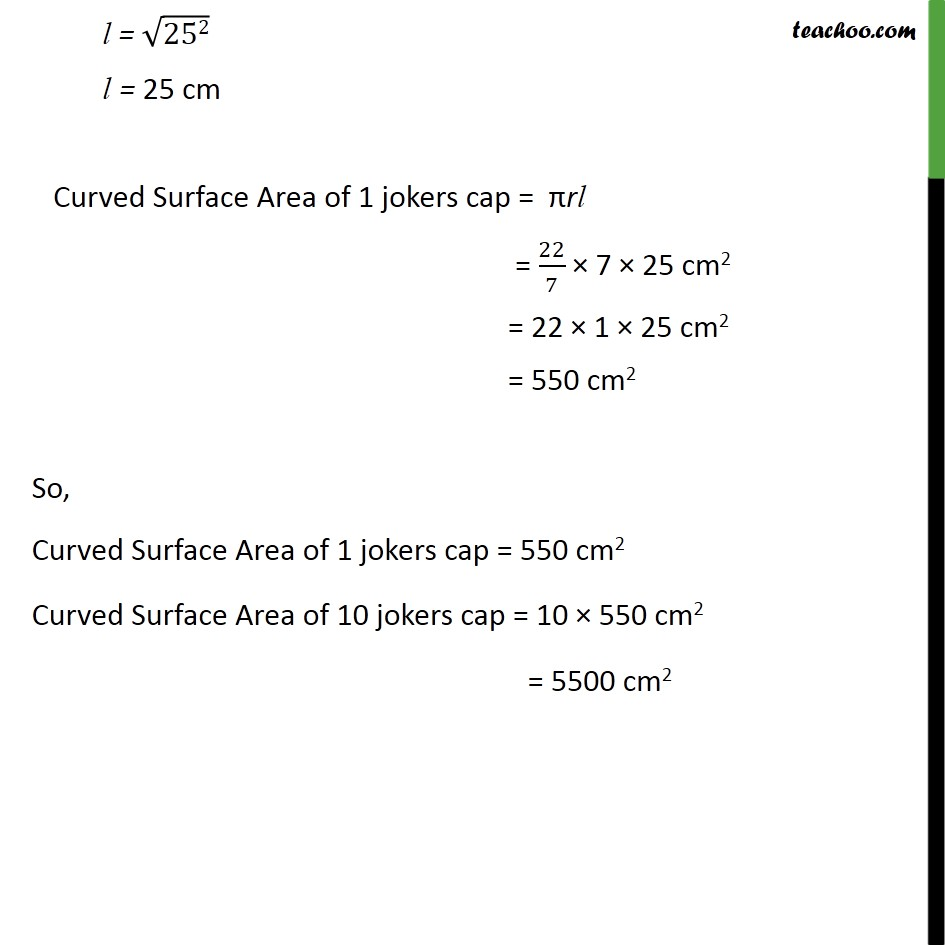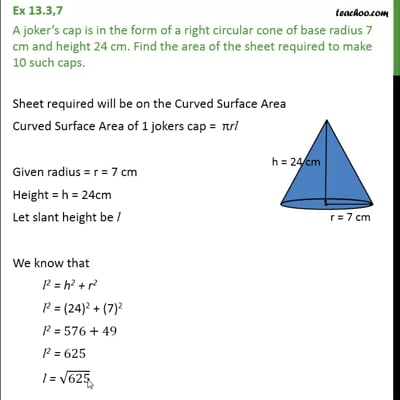Area Of Cone

Chapter 13 Class 9 Surface Areas and Volumes
Concept wiseThis video is only available for Teachoo black users

Introducing your new favourite teacher - Teachoo Black, at only ₹83 per month

### Transcript

Ex 13.3,7 A joker’s cap is in the form of a right circular cone of base radius 7 cm and height 24 cm. Find the area of the sheet required to make 10 such caps. Sheet required will be on the Curved Surface Area Curved Surface Area of 1 jokers cap = πrl Given radius = r = 7 cm Height = h = 24cm Let slant height be l We know that l2 = h2 + r2 l2 = "(24)2 + (7)2" l2 = 576+49 l2 = 625 l = √625 l = √("252" ) l = 25 cm Curved Surface Area of 1 jokers cap = πrl = 22/7 × 7 × 25 cm2 = 22 × 1 × 25 cm2 = 550 cm2 So, Curved Surface Area of 1 jokers cap = 550 cm2 Curved Surface Area of 10 jokers cap = 10 × 550 cm2 = 5500 cm2